[Next] [Up] [Previous] [Index]
Main : Index : Pencil and Paper Systems : Methods of Transposition

Methods of Transposition

After looking at ciphers which can replace the letters of one's message by completely different letters, a cipher that can't change any letters at all seems weak.

And, if your message might mention, or might not mention, someone with, say, a Q or an X in his name, then a transposition cipher will indeed give that away, although one could solve that by adding some garbage to the end of your message before encrypting it. But transposition ciphers can be secure in themselves, and as well, transposition methods are useful to know, since they can be mixed with substitution methods for a more secure cipher.

The best known method of transposition, simple columnar transposition, works like this:

Using a key word or phrase, such as CONVENIENCE, assign a number to each letter in the word using this rule: the numbers are assigned starting with 1, and they are assigned first by alphabetical order, and second, where the same letter appears twice, by position in the word.

Then, write in the message under the keyword, writing across - but take out columns, in numerical order, reading down. Thus:

C  O  N  V  E  N  I  E  N  C  E
1 10  7 11  3  8  6  4  9  2  5
---------------------------------
H  E  R  E  I  S  A  S  E  C  R
E  T  M  E  S  S  A  G  E  E  N
C  I  P  H  E  R  E  D  B  Y  T
R  A  N  S  P  O  S  I  T  I  O
N

produces

HECRN CEYI ISEP SGDI RNTO AAES RMPN
SSRO EEBT ETIA EEHS

Of course, it wouldn't be left with the spaces showing the columns that were used.

Decoding is harder - to read a message scrambled this way, you first have to count the letters to determine, in this case, that there are 45 letters, and so the first column will have five letters in it, and the other ones four, so that you know when to stop when filling the letters in vertically to read them out horizontally.

Since the text being transposed is split into nearly regular divisions of almost equal length, even double columnar transposition can be broken without recourse to multiple anagramming: the use of several messages of the same length, enciphered in the same key, to recover the transposition by matching together columns of letters that form reasonable letter pairs.

Another method of transposition, which appeared in a book by General Luigi Sacco, is a variant of columnar transposition that produces a different cipher:

C  O  N  V  E  N  I  E  N  C  E
1 10  7 11  3  8  6  4  9  2  5
---------------------------------
H
E  R  E  I  S  A  S  E  C  R
E  T  M  E  S
S  A  G  E  E  N  C  I
P  H  E  R  E  D  B  Y  T  R  A
N  S  P  O  S  I  T
I  O  N

produces

HEESPNI RR SSEES EIY A SCBT
EMGEPN ANDI CT RTAHSO IEERO

Here, the first row is filled in only up to the column with the key number 1; the second row is filled in only up to the column with the key number 2; and so on. Of course, one still stops when one runs out of plaintext, so the eighth row stops short of the key number 8 in this example. This method has the advantage of dividing the text being transposed in a more irregular fashion than ordinary columnar transposition.

Various methods of modifying ordinary columnar transposition slightly to make it irregular have been used from time to time.

For example, during World War I, the French army used a transposition in which diagonal lines of letters were read off before the rest of the diagram. Also, several countries have used columnar transpositions in which several positions in the grid were blanked out and not used.

The method of transposition used on the digits produced by a straddling checkerboard in the VIC cipher can be illustrated here with the alphabet; first, knowing the number of letters to be encrypted, the area to be filled is laid out, and then the triangular areas on the right to be filled with plaintext last are marked out:

2 4 3 1 5
-----------
a b c d e
f g h i U
j k l V W
m n X Y Z
o p q r s
t
here, the alphabet becomes DIVYR AFJMOT CHLXQ BGKNP EUWZS.

Another interesting form of transposition is the "turning grille", used by Germany during the First World War.

A square grille, divided into a grid of squares, one-quarter of which are punched with holes, is placed over a sheet of paper. The message is written on the paper through the holes, and then the grille is rotated by 90 degrees, and then the message continues to be written, as the grille is turned through all four possible positions.

The trick to designing such a grille is to divide the grille into quarters, numbering the squares in each quarter so that as the grille is rotated, corresponding squares have the same number. Then, choose one square with each number for punching.

In World War I, the Germans used turning grilles with an odd number of holes on each side as well as with an even number; to do this, they marked the square in the centre, which was always punched, with a black border to indicate it was only to be used in one of the grille's positions.

Example of a turning grille and its use:

Grid numbering:

1  2  3  4  5 16 11  6  1
6  7  8  9 10 17 12  7  2
11 12 13 14 15 18 13  8  3
16 17 18 19 20 19 14  9  4
5 10 15 20  X 20 15 10  5
4  9 14 19 20 19 18 17 16
3  8 13 18 15 14 13 12 11
2  7 12 17 10  9  8  7  6
1  6 11 16  5  4  3  2  1

Layout:

O  -  -  O  -  O  -  -  -   1        4    16
-  -  O  -  -  -  -  -  O         8                 2
-  O  -  -  -  -  O  -  -     12             13
-  -  O  -  O  -  -  O  -        18    20        9
-  -  -  -  X  -  O  -  O               X    15     5
-  -  -  O  -  -  -  O  -           19          17
O  -  -  -  -  O  -  -  -   3             14
-  O  -  -  O  -  -  -  O      7       10           6
-  -  O  -  -  -  -  -  -        11

Filling-in:

first position
T        H     I            O  -  -  O  -  O  -  -  -
S                 I   -  -  O  -  -  -  -  -  O
S              A         -  O  -  -  -  -  O  -  -
M     E        S      -  -  O  -  O  -  -  O  -
S     A     G   -  -  -  -  O  -  O  -  O
E           T      -  -  -  O  -  -  -  O  -
H              A            O  -  -  -  -  O  -  -  -
T        I           A   -  O  -  -  O  -  -  -  O
M                     -  -  O  -  -  -  -  -  -
(this is a message that I am)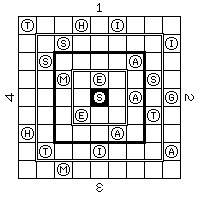second position
t  E     h  N  i     C      -  O  -  -  O  -  -  O  -
s  R     Y        i   -  -  -  O  -  O  -  -  -
s  P     T     a         -  -  O  -  O  -  -  -  -
I     m     e     N  s      O  -  -  -  -  -  O  -  -
G  s     a  W  g   -  -  -  O  .  -  -  O  -
I        e     T     t      O  -  -  -  -  O  -  -  -
h  H     A     a        T   -  O  -  O  -  -  -  -  O
t  U     i        R  a   -  -  O  -  -  -  -  O  -
N     m           I         O  -  -  -  -  -  O  -  -
(encrypting with a turni)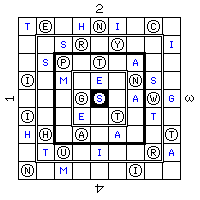third position
t  e     h  n  i  N  c      -  -  -  -  -  -  O  -  -
G     s  r  G  y     R  i   O  -  -  -  O  -  -  O  -
s  p  I  t     a     L   -  -  -  O  -  -  -  -  O
i  L  m     e  E  n  s      -  O  -  -  -  O  -  -  -
T     O  g  s     a  w  g   O  -  O  -  .  -  -  -  -
i  P     e  R  t  O  t      -  O  -  -  O  -  O  -  -
h  h  V  a     a     I  t   -  -  O  -  -  -  -  O  -
D  t  u     i     E  r  a   O  -  -  -  -  -  O  -  -
n     m  T     H  i     I   -  -  -  O  -  O  -  -  O
(ng grille to provide thi)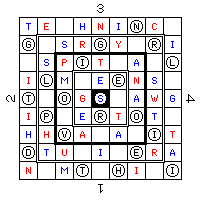fourth position
t  e  S  h  n  i  n  c  I   -  -  O  -  -  -  -  -  O
g  L  s  r  g  y  L  r  i   -  O  -  -  -  -  O  -  -
U  s  p  i  t  S  a  T  l   O  -  -  -  -  O  -  O  -
i  l  m  R  e  e  n  s  A   -  -  -  O  -  -  -  -  O
t  T  o  g  s  I  a  w  g   -  O  -  -  .  O  -  -  -
i  p  V  e  r  t  o  t  E   -  -  O  -  -  -  -  -  O
h  h  v  a  E  a  X  i  t   -  -  -  -  O  -  O  -  -
d  t  i  A  i  M  e  r  a   -  -  -  O  -  O  -  -  -
n  P  m  t  L  h  i  E  i   -  O  -  -  O  -  -  O  -
(s illustrative example)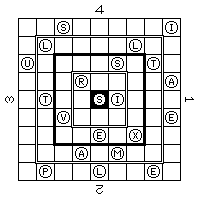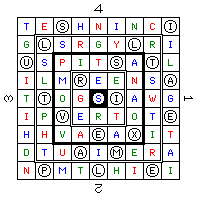to produce the encrypted result:

TESHN INCIG LSRGY LRIUS PITSA TLILM
REENS AITOG SIAWG IPVER TOTEH HVAEA
XITDT IAIME RANPM TLHIE I

There are two important uses of transposition which are connected with substitution ciphers.

Transposition can be used to generate a scrambled order of the letters in the alphabet for use as a substitution alphabet.

Transposition forms part of a fractionation cipher, where letters are divided into parts, then the parts are put back together in a different order, belonging to different letters.

[Next] [Up] [Previous] [Index]